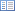Code of Federal Regulations (Last Updated: April 25, 2019)Title 40 - Protection of EnvironmentCHAPTER I — ENVIRONMENTAL PROTECTION AGENCYSUBCHAPTER C — AIR PROGRAMSPart 60 - STANDARDS OF PERFORMANCE FOR NEW STATIONARY SOURCESSubpart WW — Standards of Performance for the Beverage Can Surface Coating Industry

# § 60.493 Performance test and compliance provisions.

• (a) Section 60.8(d) does not apply to monthly performance tests and §60.8(f) does not apply to the performance test procedures required by this subpart.

(b) The owner or operator of an affected facility shall conduct an initial performance test as required under §60.8(a) and thereafter a performance test each calendar month for each affected facility.

(1) The owner or operator shall use the following procedures for each affected facility that does not use a capture system and a control device to comply with the emission limit specified under §60.492. The owner or operator shall determine the VOC-content of the coatings from formulation data supplied by the manufacturer of the coating or by an analysis of each coating, as received, using Method 24. The Administrator may require the owner or operator who uses formulation data supplied by the manufacturer of the coating to determine the VOC content of coatings using Method 24 or an equivalent or alternative method. The owner or operator shall determine from company records the volume of coating and the mass of VOC-solvent added to coatings. If a common coating distribution system serves more than one affected facility or serves both affected and exiting facilities, the owner or operator shall estimate the volume of coating used at each facility by using the average dry weight of coating, number of cans, and size of cans being processed by each affected and existing facility or by other procedures acceptable to the Administrator.

(i) Calculate the volume-weighted average of the total mass of VOC per volume of coating solids used during the calendar month for each affected facility, except as provided under paragraph (b)(1)(iv) of this section. The volume-weighted average of the total mass of VOC per volume of coating solids used each calendar month will be determined by the following procedures.

(A) Calculate the mass of VOC used (Mo + Md) during the calendar month for the affected facility by the following equation:(B) Calculate the total volume of coating solids used (Ls) in the calendar month for the affected facility by the following equation:(C) Calculate the volume-weighted average mass of VOC per volume of solids used (G) during the calendar month for the affected facility by the following equation:(ii) Calculate the volume-weighted average of VOC emissions discharged to the atmosphere (N) during the calendar month for the affected facility by the following equation:(iii) Where the value of the volume-weighted average mass of VOC per volume of solids discharged to the atmosphere (N) is equal to or less than the applicable emission limit specified under §60.492, the affected facility is in compliance.

(iv) If each individual coating used by an affected facility has a VOC content equal to or less than the limit specified under §60.492, the affected facility is in compliance provided no VOC-solvents are added to the coating during distribution or application.

(2) An owner or operator shall use the following procedures for each affected facility that uses a capture system and a control device that destroys VOC (e.g., incinerator) to comply with the emission limit specified under §60.492.

(i) Determine the overall reduction efficiency (R) for the capture system and control device.

(A) Determine the fraction (F) of total VOC used by the affected facility that enters the control device using the following equation:(B) Determine the destruction efficiency of the control device (E) using values of the volumetric flow rate of each of the gas streams and the VOC content (as carbon) of each of the gas streams in and out of the device by the following equation:(C) Determine overall reduction efficiency (R) using the following equation:(ii) Calculate the volume-weighted average of the total mass of VOC per volume of coating solids (G) used during the calendar month for the affected facility using equations (1), (2), and (3).

(iii) Calculate the volume-weighted average of VOC emissions discharged to the atmosphere (N) during the calendar month by the following equation:(iv) If the volume-weighted average of mass of VOC emitted to the atmosphere for the calendar month (N) is equal to or less than the applicable emission limit specified under §60.492, the affected facility is in compliance.

(3) An owner or operator shall use the following procedure for each affected facility that uses a capture system and a control device that recovers the VOC (e.g., carbon adsorber) to comply with the applicable emission limit specified under §60.492.

(i) Calculate the volume-weighted average of the total mass of VOC per unit volume of coating solids applied (G) used during the calendar month for the affected facility using equations (1), (2), and (3).

(ii) Calculate the total mass of VOC recovered (Mr) during each calendar month using the following equation:(iii) Calculate overall reduction efficiency of the control device (R) for the calendar month for the affected facility using the following equation: